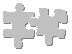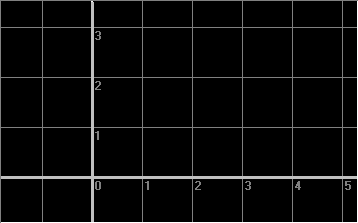#Why A Graph?[Why A Graph?]

We've learned that phi + 1 = phi * phi, but what does that have to do with graphs and solutions to equations?

First off, let's observe convention: In the language of mathematics, we will be working with a variable we'll call 'x', the unknown. We want to find a value for x that makes our equation true. When that happens (if it happens), the number that x equals at that time will be phi. So let's start by restating our equation as: x + 1 = x * x.

An equation is a statement that shows a relationship between two sets of numbers. It states that, under the conditions it describes, the expression on the left side of the '=' sign has the same value as the expression on the right side.

A special type of equation is the function. A function says that if you perform the calculations ('evaluate the expression') described on the right-hand side of the '=' sign, you will get some result. By convention, we generally call the number you perform the calculations on 'x' and the result of the calculations 'y'.

The simplest functions just return a constant value; 'y = 1' is an example. This says that, no matter what value x takes on, y will always be 1. Well, so what? x isn't even in the equation.

Things get more interesting when you bring in x. For instance, 'y = x' says that no matter what value you give x, y will be equal to the same number. Or, remembering simple geometry, 'y = pi * x' tells you that, in a circle, if you take the value of the diameter (x) and multiply it by pi, the result you get in y will be the circumference of the circle, no matter what diameter circle you're talking about.

There are two ways we usually solve such equations. One is to use algebra, which gives more accurate results but at the expense of only yielding up a number.

The other is through the use of graphs, which don't give as accurate a solution but show what a function 'looks' like.

So let's go back to our equation. It says that there exists some number ('x') such that when you add 1 to it you get the same number as when you multiply it by itself. Wait a minute - we're performing calculations and getting results here; isn't that what functions do? The equation really says that two functions return the same number, doesn't it? Let's rewrite it as two functions,

y = x + 1
y = x * x

A while back a man named Descartes realized that you could take a function and, instead of solving it for just one value, solve it for a whole range of values. You imagine that every x corresponds to a point on a horizontal line (the 'x axis'), and every y to a point on a vertical line (the 'y axis'). Then, for every x on the horizontal line, you calculate the associated y, move vertically from the horizontal line, and mark a point. With any luck, when you're done the result is like a connect-the-dots drawing of the function.

What happens when we graph our functions?

[More_Graphics] [Next_>_]